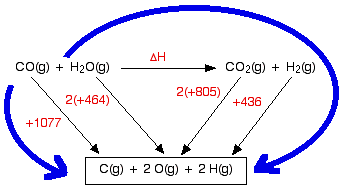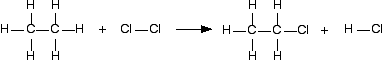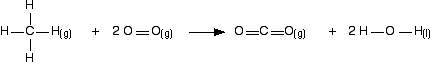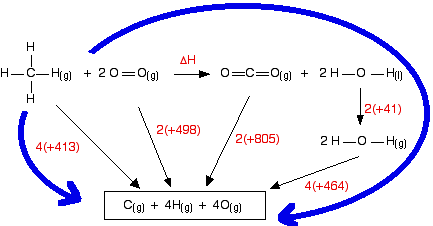Need help? Email help@shout.education

# Bond Enthalpy (Bond Energy)

This page introduces bond enthalpies (bond energies) and looks at some simple calculations involving them.

One of the most confusing things about this is the way the words are used. These days, the term "bond enthalpy" is normally used, but you will also find it described as "bond energy" – sometimes in the same article. An even older term is "bond strength". So you can take all these terms as being interchangeable.

As you will see below, though, "bond enthalpy" is used in several different ways, and you might need to be careful about this.

Note: Bond enthalpies quoted from different sources often vary by a few kilojoules, even if they are referring to exactly the same thing. Don't worry if you come across slightly different values. As you will see later on this page, calculations involving bond enthalpies hardly ever give accurate answers anyway.

## Explaining the Terms

### Bond Dissociation Enthalpy and Mean Bond Enthalpy

#### Simple Diatomic Molecules

A diatomic molecule is one that only contains two atoms. They could be the same (for example, Cl2) or different (for example, HCl).

The bond dissociation enthalpy is the energy needed to break one mole of the bond to give separated atoms – everything being in the gas state.

Important! The point about everything being in the gas state is essential. You cannot use bond enthalpies to do calculations directly from substances starting in the liquid or solid state.

As an example of bond dissociation enthalpy, to break up 1 mole of gaseous hydrogen chloride molecules into separate gaseous hydrogen and chlorine atoms takes 432 kJ. The bond dissociation enthalpy for the H-Cl bond is +432 kJ mol-1.

#### More complicated molecules

What happens if the molecule has several bonds, rather than just 1?

Consider methane, CH4. It contains four identical C-H bonds, and it seems reasonable that they should all have the same bond enthalpy.

However, if you took methane to pieces one hydrogen at a time, it needs a different amount of energy to break each of the four C-H bonds. Every time you break a hydrogen off the carbon, the environment of those left behind changes. And the strength of a bond is affected by what else is around it.

In cases like this, the bond enthalpy quoted is an average value.

In the methane case, you can work out how much energy is needed to break a mole of methane gas into gaseous carbon and hydrogen atoms. That comes to +1662 kJ and involves breaking 4 moles of C-H bonds. The average bond energy is therefore +1662/4 kJ, which is +415.5 kJ per mole of bonds.

That means that many bond enthalpies are actually quoted as mean (or average) bond enthalpies, although it might not actually say so. Mean bond enthalpies are sometimes referred to as "bond enthalpy terms".

In fact, tables of bond enthalpies give average values in another sense as well, particularly in organic chemistry. The bond enthalpy of, say, the C-H bond varies depending on what is around it in the molecule. So data tables use average values which will work well enough in most cases.

That means that if you use the C-H value in some calculation, you can't be sure that it exactly fits the molecule you are working with. So don't expect calculations using mean bond enthalpies to give very reliable answers.

You may well have to know the difference between a bond dissociation enthalpy and a mean bond enthalpy, and you should be aware that the word mean (or average) is used in two slightly different senses. But for calculation purposes, it isn't something you need to worry about. Just use the values you are given.

Important: The rest of this page assumes that you have already read the page about Hess's Law and enthalpy change calculations. If you have come straight to the current page from a search engine, you won't make sense of the way the calculations are set out unless you first read the Hess's Law page.

## Finding Enthalpy Changes of Reaction From Bond Enthalpies

I can only give a brief introduction here, because this is covered in careful, step-by-step detail in my chemistry calculations book.

### Cases Where Everything Present is Gaseous

Remember that you can only use bond enthalpies directly if everything you are working with is in the gas state.

#### Using the same method as for other enthalpy sums

We are going to estimate the enthalpy change of reaction for the reaction between carbon monoxide and steam. This is a part of the manufacturing process for hydrogen.

\text{CO}_{(g)} + \text{H}_2\text{O}_{(g)} \longrightarrow \text{CO}_{2(g)} + \text{H}_{2(g)}

The bond enthalpies are:

bond enthalpy
/ kJ mol-1
C-O in carbon monoxide+1077
C-O in carbon dioxide+805
O-H+464
H-H+436

So let's do the sum. Here is the cycle – make sure that you understand exactly why it is the way it is.And now equate the two routes, and solve the equation to find the enthalpy change of reaction.

\begin{aligned} \Delta H + (2 \times 805) + 436 &= 1077 + (2 \times 464) \\ \Delta H &= 1077 + (2 \times 464) - (2 \times 805) - 436 \\ \Delta H &= {-}41 \text{ kJ mol}^{-1} \end{aligned}

#### Using a short-cut method for simple cases

You could do any bond enthalpy sum by the method above – taking the molecules completely to pieces and then remaking the bonds. If you are happy doing it that way, just go on doing it that way.

However, if you are prepared to give it some thought, you can save a bit of time – although only in very simple cases where the changes in a molecule are very small.

For example, chlorine reacts with ethane to give chloroethane and hydrogen chloride gases.(All of these are gases. I have left the state symbols out this time to avoid cluttering the diagram.)

It is always a good idea to draw full structural formulae when you are doing bond enthalpy calculations. It makes it much easier to count up how many of each type of bond you have to break and make.

If you look at the equation carefully, you can see what I mean by a "simple case". Hardly anything has changed in this reaction. You could work out how much energy is needed to break every bond, and how much is given out in making the new ones, but quite a lot of the time, you are just remaking the same bond.

All that has actually changed is that you have broken a C-H bond and a Cl-Cl bond, and made a new C-Cl bond and a new H-Cl bond. So you can just work those out.

bond enthalpy
/ kJ mol-1
C-H+413
Cl-Cl+243
C-Cl+346
H-Cl+432

Work out the energy needed to break C-H and Cl-Cl:

413 + 243 = {+}656 \text{ kJ mol}^{-1}

Work out the energy released when you make C-Cl and H-Cl:

{-}346 - 432 = {-}778 \text{ kJ mol}^{-1}

So the net change is +656 – 778 = -122 kJ mol-1

Note: Even if you choose not to use this method, it might be a good idea to be aware of it. It is possible to imagine an examiner setting a question which assumes that you will use this method, and therefore doesn't give a particular bond enthalpy value that you would need if you did it by the longer method. For example, in the case above, you don't actually need to know the C-C bond enthalpy.

### Cases Where You Have a Liquid Present

I have to keep on saying this! Remember that you can only use bond enthalpies directly if everything you are working with is in the gas state.

If you have one or more liquids present, you need an extra energy term to work out the enthalpy change when you convert from liquid to gas, or vice versa. That term is the enthalpy change of vaporisation, and is given the symbol ΔHvap or ΔHv.

This is the enthalpy change when 1 mole of the liquid converts to gas at its boiling point with a pressure of 1 bar (100 kPa).

(Older sources might quote 1 atmosphere rather than 1 bar.)

For water, the enthalpy change of vaporisation is +41 kJ mol-1. That means that it take 41 kJ to change 1 mole of water into steam. If 1 mole of steam condenses into water, the enthalpy change would be -41 kJ. Changing from liquid to gas needs heat; changing gas back to liquid releases exactly the same amount of heat.

To see how this fits into bond enthalpy calculations, we will estimate the enthalpy change of combustion of methane – in other words, the enthalpy change for this reaction:Notice that the product is liquid water. You cannot apply bond enthalpies to this. You must first convert it into steam. To do this you have to supply 41 kJ mol-1.

The bond enthalpies you need are:

bond enthalpy
/ kJ mol-1
C-H+413
O=O+498
C=O in carbon dioxide+805
O-H+464

The cycle looks like this:This obviously looks more confusing than the cycles we've looked at before, but apart from the extra enthalpy change of vaporisation stage, it isn't really any more difficult. Before you go on, make sure that you can see why every single number and arrow on this diagram is there.

In particular, make sure that you can see why the first 4 appears in the expression "4(+464)". That is an easy thing to get wrong. (In fact, when I first drew this diagram, I carelessly wrote 2 instead of 4 at that point!)

That's the hard bit done – now the calculation:

\begin{gathered} \Delta H + (2 \times 805) + (2 \times 41) + (4 \times 464) = (4 \times 413) + (2 \times 498) \\ \\ \Delta H = (4 \times 413) + (2 \times 498) - (2 \times 805) - (2 \times 41) - (4 \times 464) \\ \\ \Delta H = {-}900 \text{ kJ mol}^{-1} \end{gathered}

The measured enthalpy change of combustion is -890 kJ mol-1, and so this answer agrees to within about 1%. As bond enthalpy calculations go, that's a pretty good estimate.

Note: Because this is all covered in more detail in my calculations book, I am afraid that this is as far as I am prepared to go with this topic. The book will give you a lot more examples, including some variations such as calculating bond enthalpies from enthalpies of formation, and vice versa.